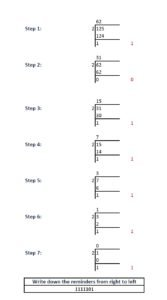# How to Convert Decimal number to Binary

## Decimal Number

Whole number, a decimal point and a fractional value combines to form a decimal number. The decimal point separates the whole number part from the fractional part of the number. Each digit of a decimal number can be any number from 0 to 9. Any value less than 1 is written to the right of decimal point. Decimal numbers are also known as base-10 number or counting numbers. Place value of decimal number varies as the whole number powers of 10 starting from the left of decimal point. Similarly, the place value of digits left to decimal point varies as the division of power of tens.

## Binary Number

Binary numbering system uses only two symbols 0 and 1. Each digits of a binary number is referred to as bits. Binary system is also known as base -2 system. Each digit is represented by the increasing power of 2 from the LSB (Least Significant Bit). Binary system is the heart of digital electronics and is used for information flow. In digital electronics, 0 and 1 are used to denote logic states, high and low. Arithmetic operations are also possible in binary system.

## How to convert decimal to binary:

To convert any decimal number to binary follow the below steps:

### Step 1:

Divide the number by 2 and note down the quotient and reminder. Let it be reminder-1 and quotient-1 respectively.

### Step 2:

Again divide the quotient-1 by 2 and note down the quotient and remainder as reminder-2 and quotient-2.

### Step 3:

Repeat Step-1 and Step-2 until the quotient becomes zero.

### Step 4:

Assume you have done the division ‘n’ times until you got a quotient of 0. From the first reminder, write down all the reminders from right to left.

Here you go. You have converted the decimal number.

## Example:Decimal number to a Binary number conversion

Let us convert 125 to binary: